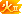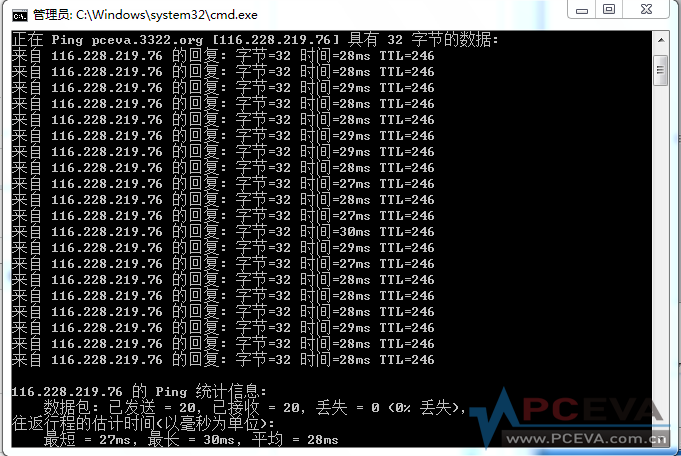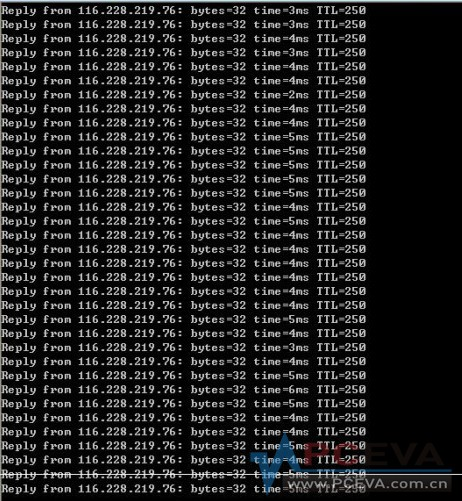# 趁着联通骨干网故障，帮忙做个测试~~~贴出结果的都有分哦[复制链接]
 C:\Users\Chris>ping bbs.pceva.com.cn -n 20 正在 Ping diablofc.3322.org [116.228.219.76] 具有 32 字节 来自 116.228.219.76 的回复: 字节=32 时间=165ms TTL=240 来自 116.228.219.76 的回复: 字节=32 时间=163ms TTL=240 来自 116.228.219.76 的回复: 字节=32 时间=163ms TTL=240 来自 116.228.219.76 的回复: 字节=32 时间=162ms TTL=240 来自 116.228.219.76 的回复: 字节=32 时间=163ms TTL=240 来自 116.228.219.76 的回复: 字节=32 时间=162ms TTL=240 来自 116.228.219.76 的回复: 字节=32 时间=164ms TTL=240 来自 116.228.219.76 的回复: 字节=32 时间=164ms TTL=240 来自 116.228.219.76 的回复: 字节=32 时间=163ms TTL=240 来自 116.228.219.76 的回复: 字节=32 时间=162ms TTL=240 来自 116.228.219.76 的回复: 字节=32 时间=162ms TTL=240 来自 116.228.219.76 的回复: 字节=32 时间=164ms TTL=240 来自 116.228.219.76 的回复: 字节=32 时间=166ms TTL=240 来自 116.228.219.76 的回复: 字节=32 时间=164ms TTL=240 来自 116.228.219.76 的回复: 字节=32 时间=162ms TTL=240 来自 116.228.219.76 的回复: 字节=32 时间=165ms TTL=240 来自 116.228.219.76 的回复: 字节=32 时间=163ms TTL=240 来自 116.228.219.76 的回复: 字节=32 时间=158ms TTL=240 来自 116.228.219.76 的回复: 字节=32 时间=164ms TTL=240 来自 116.228.219.76 的回复: 字节=32 时间=163ms TTL=240 116.228.219.76 的 Ping 统计信息:     数据包: 已发送 = 20，已接收 = 20，丢失 = 0 (0% 丢失)， 往返行程的估计时间(以毫秒为单位):     最短 = 158ms，最长 = 166ms，平均 = 163ms ----------------------------------------------------------------- 河南联通100M

### 评分gmx168 + 10 还行，在可以接受的范围内
 xiahait 发表于 2012-7-11 14:18我这边以前秒开，现在不给力呀，老湿 很正常啊，你以前是青岛连到济南，都是联通线路，都没跨省，当然秒开啦。 现在是青岛连到上海，联通到电信，又跨省，想秒开？做梦吧~~~广州电信光纤### 评分

 gmx168 发表于 2012-7-11 14:23很正常啊，你以前是青岛连到济南，都是联通线路，都没跨省，当然秒开啦。 现在是青岛连到上海，联通到电 ... 原来移到上海，难怪
 现在卡爆了，刷图都很慢，以前绝对秒开
 北京市联通 Reply from 116.228.219.76: bytes=32 time=203ms TTL=243 Reply from 116.228.219.76: bytes=32 time=202ms TTL=243 Reply from 116.228.219.76: bytes=32 time=228ms TTL=243 Reply from 116.228.219.76: bytes=32 time=205ms TTL=243 Reply from 116.228.219.76: bytes=32 time=212ms TTL=243 Reply from 116.228.219.76: bytes=32 time=202ms TTL=243 Reply from 116.228.219.76: bytes=32 time=203ms TTL=243 Reply from 116.228.219.76: bytes=32 time=194ms TTL=243 Reply from 116.228.219.76: bytes=32 time=928ms TTL=243 Reply from 116.228.219.76: bytes=32 time=201ms TTL=243 Reply from 116.228.219.76: bytes=32 time=204ms TTL=243 Reply from 116.228.219.76: bytes=32 time=213ms TTL=243 Reply from 116.228.219.76: bytes=32 time=208ms TTL=243 Reply from 116.228.219.76: bytes=32 time=210ms TTL=243 Reply from 116.228.219.76: bytes=32 time=205ms TTL=243 Reply from 116.228.219.76: bytes=32 time=207ms TTL=243 Reply from 116.228.219.76: bytes=32 time=209ms TTL=243 Reply from 116.228.219.76: bytes=32 time=204ms TTL=243 Reply from 116.228.219.76: bytes=32 time=204ms TTL=243 Reply from 116.228.219.76: bytes=32 time=204ms TTL=243 Ping statistics for 116.228.219.76:     Packets: Sent = 20, Received = 20, Lost = 0 (0% loss Approximate round trip times in milli-seconds:     Minimum = 194ms, Maximum = 928ms, Average = 242ms

### 评分gmx168 + 10 使用联通线路的会比较卡
 杭州电信10M企业光纤 正在 Ping pceva.3322.org [116.228.219.76] 具有 32 字节的数据: 来自 116.228.219.76 的回复: 字节=32 时间=17ms TTL=245 来自 116.228.219.76 的回复: 字节=32 时间=9ms TTL=245 来自 116.228.219.76 的回复: 字节=32 时间=8ms TTL=245 来自 116.228.219.76 的回复: 字节=32 时间=8ms TTL=245 来自 116.228.219.76 的回复: 字节=32 时间=7ms TTL=245 来自 116.228.219.76 的回复: 字节=32 时间=10ms TTL=245 来自 116.228.219.76 的回复: 字节=32 时间=10ms TTL=245 来自 116.228.219.76 的回复: 字节=32 时间=10ms TTL=245 来自 116.228.219.76 的回复: 字节=32 时间=10ms TTL=245 来自 116.228.219.76 的回复: 字节=32 时间=9ms TTL=245 来自 116.228.219.76 的回复: 字节=32 时间=8ms TTL=245 来自 116.228.219.76 的回复: 字节=32 时间=8ms TTL=245 来自 116.228.219.76 的回复: 字节=32 时间=8ms TTL=245 来自 116.228.219.76 的回复: 字节=32 时间=8ms TTL=245 来自 116.228.219.76 的回复: 字节=32 时间=8ms TTL=245 来自 116.228.219.76 的回复: 字节=32 时间=8ms TTL=245 来自 116.228.219.76 的回复: 字节=32 时间=8ms TTL=245 来自 116.228.219.76 的回复: 字节=32 时间=12ms TTL=245 来自 116.228.219.76 的回复: 字节=32 时间=7ms TTL=245 来自 116.228.219.76 的回复: 字节=32 时间=7ms TTL=245 116.228.219.76 的 Ping 统计信息:     数据包: 已发送 = 20，已接收 = 20，丢失 = 0 (0% 丢失)， 往返行程的估计时间(以毫秒为单位):     最短 = 7ms，最长 = 17ms，平均 = 9ms

### 评分

 kinno 发表于 2012-7-11 15:20现在卡爆了，刷图都很慢，以前绝对秒开 嗯，很标准的电信联通互联问题啊，每天总有那么几个时间段很不爽~~~这也是为啥要同时安置电信和联通双服务器互为镜像的原因了。 再等等吧，等联通线路稳定，济南服务器恢复就好了。
 今天的200分发完了，你们继续贴，我明天继续发分。
 gmx168 发表于 2012-7-11 15:30今天的200分发完了，你们继续贴，我明天继续发分。 貌似骨干网故障解决不了了……协商换IP中，但还得改备案信息石头 发表于 2012-7-11 15:43貌似骨干网故障解决不了了……协商换IP中，但还得改备案信息 我这里没问题的，你放心慢慢折腾。
 C:\Users\Administrator>ping bbs.pceva.com.cn -n 20 正在 Ping pceva.3322.org [116.228.219.76] 具有 32 字节的数据: 来自 116.228.219.76 的回复: 字节=32 时间=6ms TTL=250 来自 116.228.219.76 的回复: 字节=32 时间=5ms TTL=250 来自 116.228.219.76 的回复: 字节=32 时间=8ms TTL=250 来自 116.228.219.76 的回复: 字节=32 时间=9ms TTL=250 来自 116.228.219.76 的回复: 字节=32 时间=9ms TTL=250 来自 116.228.219.76 的回复: 字节=32 时间=5ms TTL=250 来自 116.228.219.76 的回复: 字节=32 时间=7ms TTL=250 来自 116.228.219.76 的回复: 字节=32 时间=7ms TTL=250 来自 116.228.219.76 的回复: 字节=32 时间=6ms TTL=250 来自 116.228.219.76 的回复: 字节=32 时间=24ms TTL=250 来自 116.228.219.76 的回复: 字节=32 时间=5ms TTL=250 来自 116.228.219.76 的回复: 字节=32 时间=7ms TTL=250 来自 116.228.219.76 的回复: 字节=32 时间=4ms TTL=250 来自 116.228.219.76 的回复: 字节=32 时间=12ms TTL=250 来自 116.228.219.76 的回复: 字节=32 时间=22ms TTL=250 来自 116.228.219.76 的回复: 字节=32 时间=9ms TTL=250 来自 116.228.219.76 的回复: 字节=32 时间=7ms TTL=250 来自 116.228.219.76 的回复: 字节=32 时间=5ms TTL=250 来自 116.228.219.76 的回复: 字节=32 时间=6ms TTL=250 来自 116.228.219.76 的回复: 字节=32 时间=4ms TTL=250 116.228.219.76 的 Ping 统计信息:     数据包: 已发送 = 20，已接收 = 20，丢失 = 0 (0% 丢失)， 往返行程的估计时间(以毫秒为单位):     最短 = 4ms，最长 = 24ms，平均 = 8ms 电信40m ftth

### 评分

 上海电信 30M FTTB 正常### 评分

 gmx168 发表于 2012-7-11 15:46我这里没问题的，你放心慢慢折腾。我也没问题

### 评分

 线路：广东佛山电信4M ADSL 正在 Ping pceva.3322.org [116.228.219.76] 具有 32 字节的数据 来自 116.228.219.76 的回复: 字节=32 时间=37ms TTL=246 来自 116.228.219.76 的回复: 字节=32 时间=36ms TTL=246 来自 116.228.219.76 的回复: 字节=32 时间=35ms TTL=246 来自 116.228.219.76 的回复: 字节=32 时间=36ms TTL=246 来自 116.228.219.76 的回复: 字节=32 时间=36ms TTL=246 来自 116.228.219.76 的回复: 字节=32 时间=35ms TTL=246 来自 116.228.219.76 的回复: 字节=32 时间=36ms TTL=246 来自 116.228.219.76 的回复: 字节=32 时间=36ms TTL=246 来自 116.228.219.76 的回复: 字节=32 时间=35ms TTL=246 来自 116.228.219.76 的回复: 字节=32 时间=53ms TTL=246 来自 116.228.219.76 的回复: 字节=32 时间=180ms TTL=246 来自 116.228.219.76 的回复: 字节=32 时间=37ms TTL=246 来自 116.228.219.76 的回复: 字节=32 时间=35ms TTL=246 来自 116.228.219.76 的回复: 字节=32 时间=37ms TTL=246 来自 116.228.219.76 的回复: 字节=32 时间=37ms TTL=246 来自 116.228.219.76 的回复: 字节=32 时间=44ms TTL=246 来自 116.228.219.76 的回复: 字节=32 时间=37ms TTL=246 来自 116.228.219.76 的回复: 字节=32 时间=35ms TTL=246 来自 116.228.219.76 的回复: 字节=32 时间=37ms TTL=246 来自 116.228.219.76 的回复: 字节=32 时间=35ms TTL=246 116.228.219.76 的 Ping 统计信息:     数据包: 已发送 = 20，已接收 = 20，丢失 = 0 (0% 丢失)， 往返行程的估计时间(以毫秒为单位):     最短 = 35ms，最长 = 180ms，平均 = 44ms

### 评分

 kx945` 发表于 2012-7-11 16:18我也没问题这鸟人~~~难道现在只有上海电信镜像系统在正常工作？

### 点评

 问题搞清楚了。。。。。要调整服务器设置，加什么路由表、策略路由一堆设置，菠萝又有的忙了